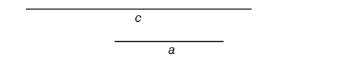Chapter 3.4, Problem 25E### Elementary Geometry for College St...

6th Edition
Daniel C. Alexander + 1 other
ISBN: 9781285195698

#### Solutions

Chapter
Section### Elementary Geometry for College St...

6th Edition
Daniel C. Alexander + 1 other
ISBN: 9781285195698
Textbook Problem
1 views

# In Exercises 23 to 26, use line segments of length a and c as shownConstruct the isosceles triangle with a vertex angle 30 ° and each leg of length cExercises 23-26

To determine

To draw:

The isosceles triangle with vertex angle 30° and each leg of length c.

Explanation

Given:

The following figure shows the given diagram.

Figure (1)

Approach:

Consider the line a and mark its end point as A and B as shown below.

Figure (2)

Take point A and B as center and draw two circle of equal radius A and mark the intersection points as shown below.

Figure (3)

Draw the two line segments AC¯ and BC¯ as shown below.

Figure (4)

In the above figure, it is clearly visible that the ΔABC is regular triangle and mA=60°.

Take point C as center and draw a circle of radius AB and mark the intersection points as shown below.

### Still sussing out bartleby?

Check out a sample textbook solution.

See a sample solution

#### The Solution to Your Study Problems

Bartleby provides explanations to thousands of textbook problems written by our experts, many with advanced degrees!

Get Started

#### In Exercises 6372, evaluate the expression. 69. | 1| + 2

Applied Calculus for the Managerial, Life, and Social Sciences: A Brief Approach

#### Convert the expressions in Exercises 8596 radical form. 34(1x)5/2

Finite Mathematics and Applied Calculus (MindTap Course List)

#### What is a ceiling effect, and how can it be a problem?

Research Methods for the Behavioral Sciences (MindTap Course List)